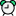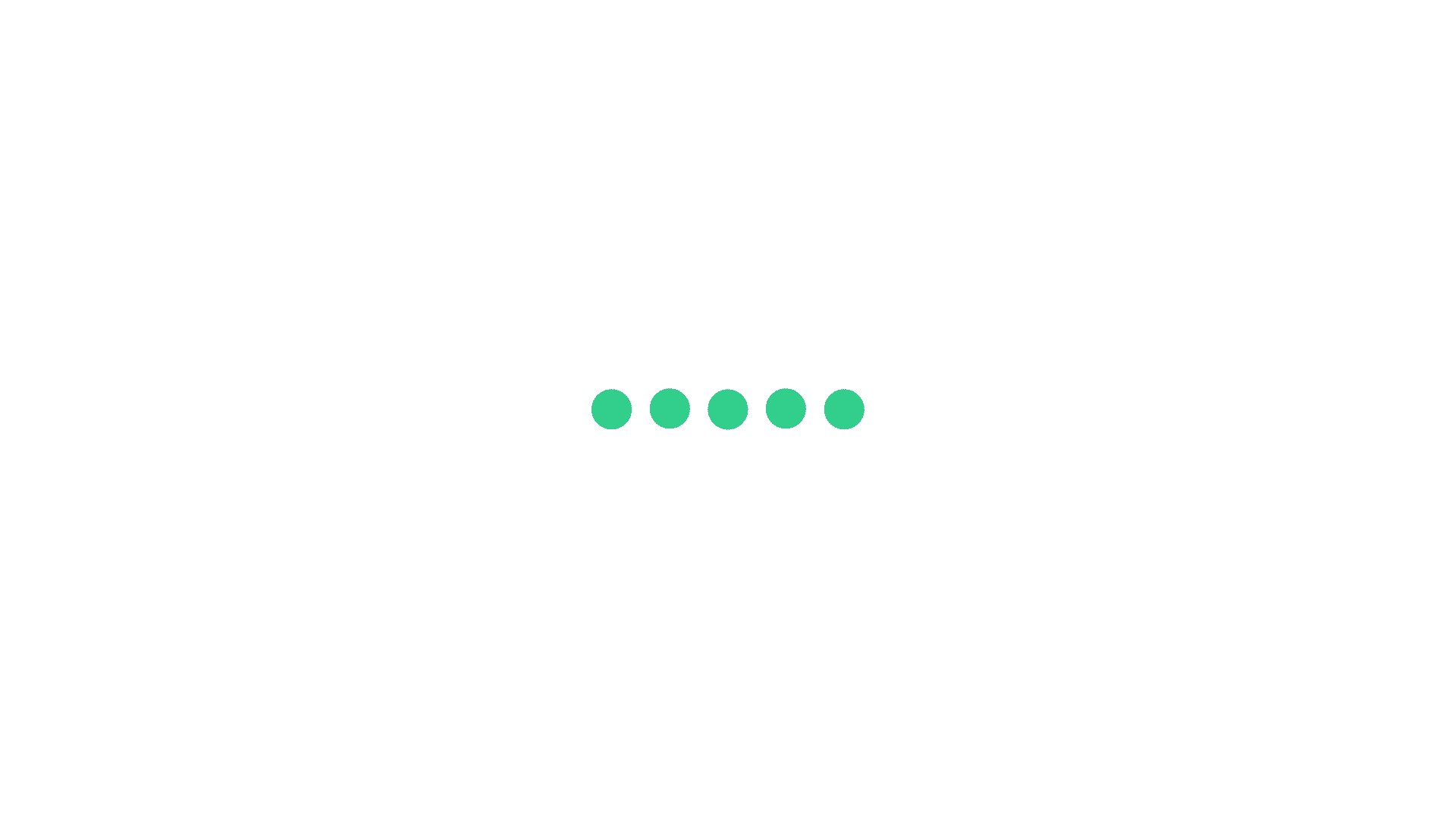# TCS Divisibility Question Answers 2

Question 1Time: 00:00:00
Sarita Wants to gift the stationary kit. Which includes a pencil, an eraser and a sharpener to some kids in her village. She went to a wholesaler for purchase these items. The seller sells pencils in packing of 12. Erasers in a packing 18 and Sharpener in a packing of 10.She purchases the minimum number of packets of pencils,eraser and sharpener.so that affix number of kits can be prepared without any item being unused or short. What is the number of packet of pencils purchased by her ?10

10

12

12

15

15

18

18

Once you attempt the question then PrepInsta explanation will be displayed.Start

Question 2Time: 00:00:00
What is the greatest four digit number divisible by 5 , 10 , 15 and 20?9910

9910

9990

9990

9980

9980

9960

9960

Once you attempt the question then PrepInsta explanation will be displayed.Start

Question 3Time: 00:00:00
What is the greatest possible positive integer n if $8^{n}$ divides $(44)^{44}$ without leaving a remainder?41

41

39

39

29

29

19

19

Once you attempt the question then PrepInsta explanation will be displayed.Start

Question 4Time: 00:00:00
Find the number of divisors of 1728.?100

100

41

41

36

36

28

28

Once you attempt the question then PrepInsta explanation will be displayed.Start

Question 5Time: 00:00:00
what is the remainder of $\frac{(16937^{30})}{31}$1

1

2

2

3

3

6

6

Once you attempt the question then PrepInsta explanation will be displayed.

16937 & 31 are coprimes so we can use Euler\'s theorem to find 16937^30 mod 31. By Euler\'s totient function, Phi (31) = 30. For a prime p, phi (p) = p-1. By Euler\'s theorem, 16937^[phi (31)] mod 31 = 1. So, 16937^30 mod 31 = 1. Read more on Brainly.in - https://brainly.in/question/5047203#readmoreStart

Question 6Time: 00:00:00
The numbers 272738 and 232342, when divided by n, a 2 digit number,  leave a remainder of 13 and 17 respectively. Find the sum of the digits of n?7

7

8

8

5

5

4

4

Once you attempt the question then PrepInsta explanation will be displayed.Start

Question 7Time: 00:00:00
What is the remainder of $32^{31^{301}}$ when it is divided by 9?3

3

5

5

2

2

1

1

Once you attempt the question then PrepInsta explanation will be displayed.Start

Question 8Time: 00:00:00
Which of the following numbers must be added to 5678 to give a reminder 35 when divided by 460?955

955

618

618

980

980

797

797

Once you attempt the question then PrepInsta explanation will be displayed.Start

Question 9Time: 00:00:00
If a number is divided by 357 the remainder is 5, what will be the remainder if the number is divided by 17?9

9

3

3

5

5

7

7

Once you attempt the question then PrepInsta explanation will be displayed.Start

Question 10Time: 00:00:00
26ab5 is a four-digit number divisible by 25. If the number formed from the two digits ab is a multiple of 13, then ab =?52

52

65

65

10

10

25

25

Once you attempt the question then PrepInsta explanation will be displayed.